#Function Repository Resource:

# KnuthBendixCompletion

Compute the Knuth-Bendix completion for a given multiway system

Contributed by: Jonathan Gorard
 ResourceFunction["KnuthBendixCompletion"][rules,init,n] generates a list of Knuth-Bendix completion rules for the multiway system with the specified rules after n steps, starting with initial conditions init. ResourceFunction["KnuthBendixCompletion"][rules→sel,init,n] uses the function sel to select which of the events obtained at each step to include in the evolution.

## Details and Options

Rules can be specified in the following ways:
 {"lhs1"->"rhs1",…} string substitution system {{l11,l12,…}->{r11,r12,..},…} list substitution system WolframModel[rules] Wolfram Model system CellularAutomaton[rules] cellular automaton system "type"→rules system of the specified type
Supported rule types include:
 "StringSubstitutionSystem" rules given as replacements on strings "ListSubstitutionSystem" rules given as replacements on lists "CellularAutomaton" rules given as a list of CellularAutomaton rule specifications "WolframModel" rules given as replacements on hypergraphs
When rules are specified by an explicit association, the following elements can be included:
 "StateEvolutionFunction" gives the list of successors to a given state "StateEquivalenceFunction" determines whether two states should be considered equivalent "StateEventFunction" gives the list of events applicable to a given state "EventApplicationFunction" applies an event to a given state "EventDecompositionFunction" decomposes an event into creator and destroyer events for individual elements "SystemType" system type name "EventSelectionFunction" determines which events should be applied to a given state
The event selection function sel in ResourceFunction["KnuthBendixCompletion"][rulessel,] can have the following special forms:
 "Sequential" applies the first possible replacement (sequential substitution system) "Random" applies a random replacement {"Random",n} applies n randomly chosen replacements "MaxScan" applies the maximal set of spatially-separated replacements (strings only)
The initial condition for ResourceFunction["KnuthBendixCompletion"] is a list of states appropriate for the type of system used.
ResourceFunction["KnuthBendixCompletion"][rules,"string",] is interpreted as ResourceFunction["KnuthBendixCompletion"][rules,{"string"},].
ResourceFunction["KnuthBendixCompletion"] accepts both individual rules and lists of rules, and likewise for initial conditions.
Options for ResourceFunction["KnuthBendixCompletion"] include:
 "IncludeStepNumber" False whether to label states and events with their respective step numbers "IncludeStateID" False whether to label states and events with unique IDs
Knuth-Bendix completion transforms an arbitrary rewrite system into a confluent (causal invariant) rewrite system by resolving critical pairs.
In the context of the multiway systems, critical (branch) pairs indicate bifurcations or ambiguities in the multiway evolution.
ResourceFunction["KnuthBendixCompletion"] gives the minimal set of rules required to force all unresolved branch pairs to converge.

## Examples

### Basic Examples (4)

Generate the list of all Knuth-Bendix completion rules for two string substitution systems:

 In:=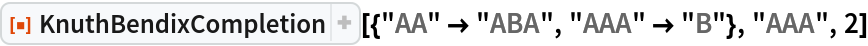Out=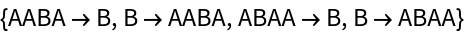In:=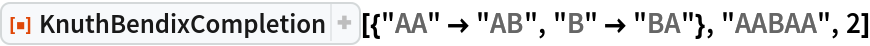Out=Different event selection functions can lead to different lists of Knuth-Bendix completion rules:

 In:=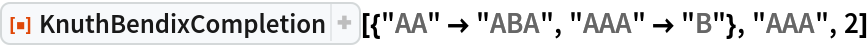Out=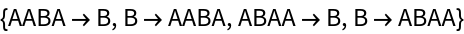In:=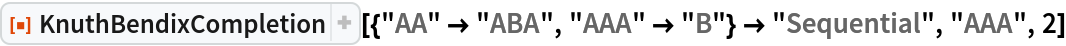Out=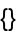KnuthBendixCompletion can handle Wolfram models and other system types:

 In:=Out=Provide a cellular automaton as input:

 In:=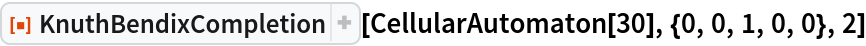Out=Preventing identical states from being merged, by including step numbers and/or state IDs, can change the resulting Knuth-Bendix completions:

 In:=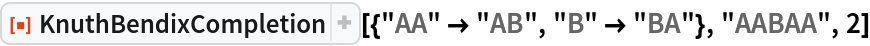Out=In:=Out=### Scope (13)

#### System Types (8)

KnuthBendixCompletion supports both string and list substitution systems:

 In:=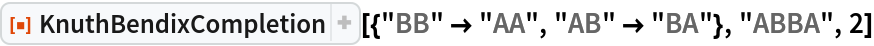Out=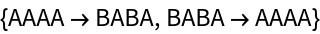Use substitutions on lists:

 In:=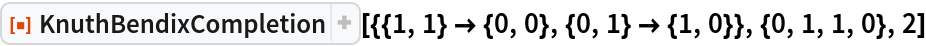Out=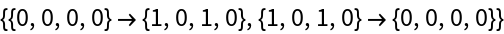Lists can contain arbitrary symbolic elements:

 In:=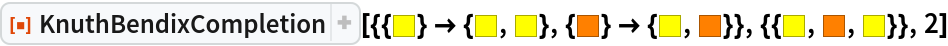Out=Give an explicit substitution system rule:

 In:=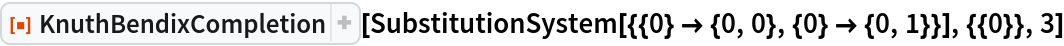Out=An alternative method of specifying that a substitution system should be used:

 In:=Out=KnuthBendixCompletion also supports multiway generalizations of cellular automata:

 In:=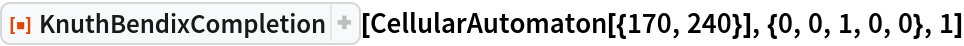Out=Generate a Knuth-Bendix completion for left- and right-shift cellular automaton rules after 3 steps:

 In:=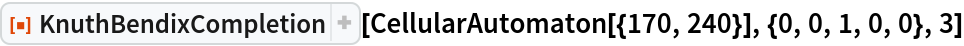Out=Determine that the rule 30 cellular automaton is not causal invariant:

 In:=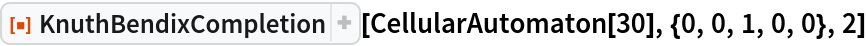Out=KnuthBendixCompletion also supports multiway generalizations of Wolfram Models:

 In:=Out=In:=Out=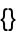Construct a multiway evolution by explicitly specifying an association:

 In:=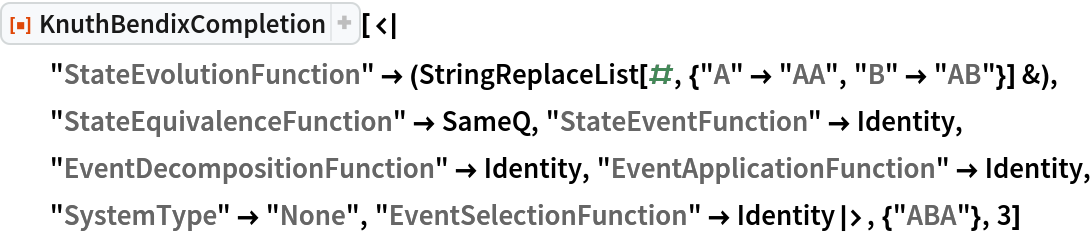Out=#### Rules and Initial Conditions (2)

KnuthBendixCompletion accepts both individual rules and lists of rules:

 In:=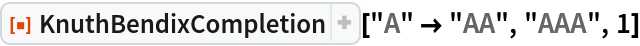Out=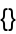In:=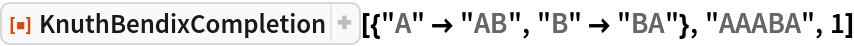Out=Likewise for initial conditions:

 In:=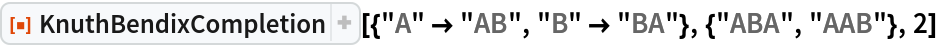Out=#### Event Selection Functions (3)

Apply only the first possible event at each step:

 In:=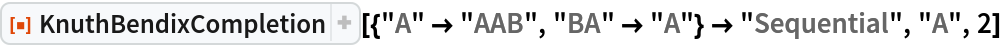Out=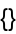Apply the first and last possible events at each step:

 In:=Out=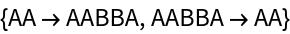Use a greedy-style algorithm to apply the maximal set of non-conflicting events at each step (strings only):

 In:=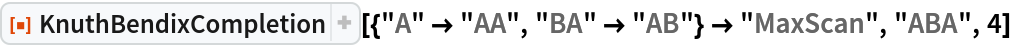Out=### Options (3)

Explicitly specify the type of rule:

 In:=Out=In:=Out=#### Step Numbers and State IDs (3)

By default, equivalent states are merged across all time steps:

 In:=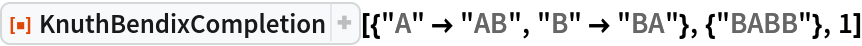Out=Merging of equivalent states across different time steps can be prevented by including step numbers:

 In:=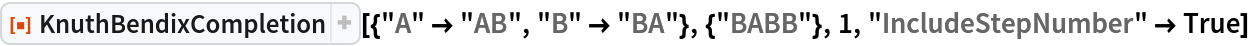Out=Merging of equivalent states at the same time step can be prevented by also including state IDs:

 In:=Out=### Applications (2)

#### Causal Invariant Rules (2)

Prove that the Xor and And functions are causal invariant (due to associativity):

 In:=Out=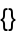In:=Out=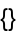On the other hand, the Nand function is not associative, so it is not total causal invariant:

 In:=Out=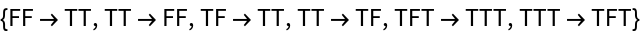### Properties and Relations (1)

KnuthBendixCompletion returning an empty list of Knuth-Bendix completion rules is a sufficient (but not necessary) condition for causal invariance:

 In:=Out=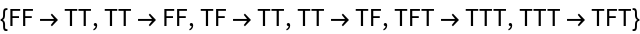In:=Out=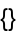## Version History

• 1.0.0 – 13 April 2020